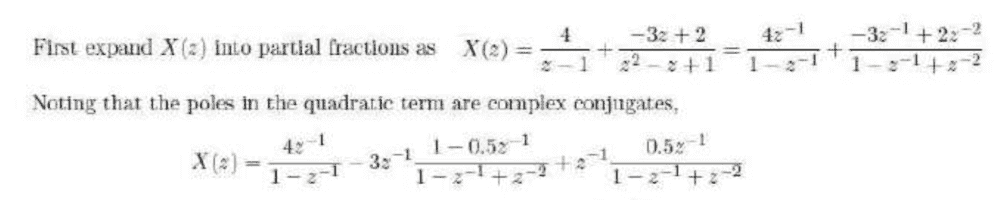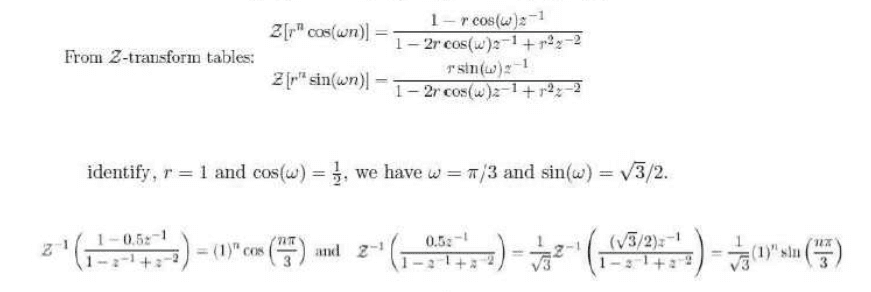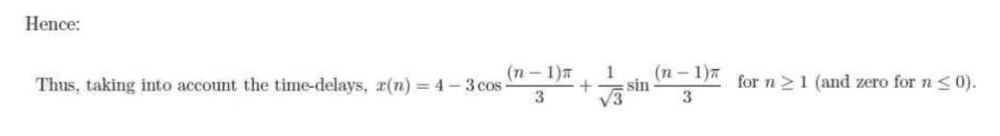# Using Complex Conjugates to Decompose a Fraction

WhiteWolf98
Homework Statement:
How do you decompose the following:

$$\frac{-3z^{-1}+2z^{-2}}{1-z^{-1}+z^{-2}}$$
Relevant Equations:
None.
So the original question is from Control Theory, and the topic is the inverse z-transform. This is a part from the solution I just can't understand. The reason it has to be in this form (##z^{-1}##) is because that's the form used in the z-transform table. The question essentially is, how do you get from the left side to the right side:

$$\frac{-3z^{-1}+2z^{-2}}{1-z^{-1}+z^{-2}}=-3z^{-1}\frac{1-0.5z^{-1}}{1-z^{-1}+z^{-2}}+z^{-1}\frac{0.5z^{-1}}{1-z^{-1}+z^{-2}}$$

In the solution, it states: 'Noting that the poles in the quadratic term are complex conjugates'. I have no idea what that means. In the normal (##z##) form, it is:

$$\frac{-3z+2}{z^2-z+1}$$

The solutions of this, I know, are complex conjugates:

$$\frac{1}{2}+\frac{\sqrt{3}}{2}i,\,\frac{1}{2}-\frac{\sqrt{3}}{2}i$$

I don't see how that helps me though. Any help would be appreciated. Thank you.

Mentor
Homework Statement:: How do you decompose the following:

$$\frac{-3z^{-1}+2z^{-2}}{1-z^{-1}+z^{-2}}$$
Relevant Equations:: None.

So the original question is from Control Theory, and the topic is the inverse z-transform. This is a part from the solution I just can't understand. The reason it has to be in this form (##z^{-1}##) is because that's the form used in the z-transform table. The question essentially is, how do you get from the left side to the right side:

$$\frac{-3z^{-1}+2z^{-2}}{1-z^{-1}+z^{-2}}=-3z^{-1}\frac{1-0.5z^{-1}}{1-z^{-1}+z^{-2}}+z^{-1}\frac{0.5z^{-1}}{1-z^{-1}+z^{-2}}$$
In the above, they rewrote the numerator of the first term as ##-3z^{-1} + 1.5z^{-2} + .5z^{-2}##.
They then made two separate fractions, with the first's numerator the first two terms above, and the second's numerator the remaining term. Finally, they factored ##-3z^{-1}## from the first fraction and ##z^{-1}## from the second.
WhiteWolf98 said:
In the solution, it states: 'Noting that the poles in the quadratic term are complex conjugates'. I have no idea what that means. In the normal (##z##) form, it is:

$$\frac{-3z+2}{z^2-z+1}$$
I haven't worked things through, but I suspect that they rewrote the common denominators like so: $$1 - z^{-1} + z^{-2} = 1 - \frac 1 z + \frac 1 {z^2}$$
and then rewrote the last expression as $$\frac{z^2 - z + 1}{z^2}$$
WhiteWolf98 said:
The solutions of this, I know, are complex conjugates:

$$\frac{1}{2}+\frac{\sqrt{3}}{2}i,\,\frac{1}{2}-\frac{\sqrt{3}}{2}i$$

I don't see how that helps me though. Any help would be appreciated. Thank you.

•WhiteWolf98
WhiteWolf98
In the above, they rewrote the numerator of the first term as −3z−1+1.5z−2+.5z−2.
Okay... that makes sense. But then the next part:
They then made two separate fractions, with the first's numerator the first two terms above, and the second's numerator the remaining term. Finally, they factored −3z−1 from the first fraction and z−1 from the second.
How can you just split a fraction like that? For example:
$$\frac{x+y}{z}=\frac{x}{z}+\frac{y}{z}$$
You can do that. But what you can't do is:
$$\frac{x+y}{w+z}=\frac{x}{w+z}+\frac{y}{w+z}$$
So how come it's being done there? Is there something I'm missing here?

I also don't see how the fact that the solutions of the denominator are complex conjugates, is being used here. I get it needs to be in a certain form, but it seems pretty random for this statement to be a reason for splitting the fraction like so. Or I'm just missing something/ there's a rule I don't know

Mentor
Okay... that makes sense. But then the next part:

How can you just split a fraction like that? For example:
$$\frac{x+y}{z}=\frac{x}{z}+\frac{y}{z}$$
You can do that. But what you can't do is:
$$\frac{x+y}{w+z}=\frac{x}{w+z}+\frac{y}{w+z}$$
No, the above is perfectly valid. You should review the basics of fraction addition.

You could also do something like this, if there's a need for it.
$$\frac{x+y}{z}=\frac{x - y}{z}+\frac{2y}{z}$$
This is similar to what they did in the work you posted.
What you can't do is the following:
$$\frac{z}{x + y}=\frac{z}{x}+\frac{z}{y}$$
WhiteWolf98 said:
So how come it's being done there? Is there something I'm missing here?

I also don't see how the fact that the solutions of the denominator are complex conjugates, is being used here. I get it needs to be in a certain form, but it seems pretty random for this statement to be a reason for splitting the fraction like so. Or I'm just missing something/ there's a rule I don't know

•WhiteWolf98
WhiteWolf98
No, the above is perfectly valid. You should review the basics of fraction addition.
Wow, uh. Right you are, my apologies. That's quite embarrassing lol

What you can't do is the following:
$$\frac{z}{x + y}=\frac{z}{x}+\frac{z}{y}$$
THAT is what I meant to say! Seems I've got it all muddled up. Thanks a lot Mark, that's made it quite clear how that fraction is split.

Homework Helper
Gold Member
I think it is simpler than that. It is easy to see the identity by rewriting the numerator as ##-3z^{-1}+1.5z^{-2}-.5z^{-2}## and separating the fraction into two fractions. I do not see how any of this has anything to do with the poles being complex conjugates. You should complete the statement in the solution. What does it say after 'Noting that the poles in the quadratic term are complex conjugates'? (Or perhaps this is the motivation for deciding to rewrite the fraction as they did?)

WhiteWolf98
I think it is simpler than that. It is easy to see the identity by rewriting the numerator as ##-3z^{-1}+1.5z^{-2}-.5z^{-2}## and separating the fraction into two fractions. I do not see how any of this has anything to do with the poles being complex conjugates. You should complete the statement in the solution. What does it say after 'Noting that the poles in the quadratic term are complex conjugates'? (Or perhaps this is the motivation for deciding to rewrite the fraction as they did?)That's the solution as I saw it; everything after is using the inverse z-transform. And I agree, I still have absolutely no clue what complex conjugates have to do with this

Homework Helper
consider the familiar Z-transform pair
$$(1-r e^{\pi i t}z^{-1})^{-1}\leftrightarrow r^n e^{n\pi i t}\mathrm{u}(n)$$
if a real to real function has a complex singularity it must have the conjugate as well. When the above pair appears so to will its conjugate
$$(1-r e^{-\pi i t}z^{-1})^{-1}\leftrightarrow r^n e^{-n\pi i t}\mathrm{u}(n)$$
the sum of the above two pairs divided by 2 being
$$\frac{1-r\cos(\pi t)z^{-1}}{1-2r \cos(\pi t)z^{-1}+r^2z^{-2}}\leftrightarrow r^n \cos(n\pi t)\mathrm{u}(n)$$
and the difference divided by 2
$$\frac{r\sin(\pi t)z^{-1}}{1-2r \cos(\pi t)z^{-1}+r^2z^{-2}}\leftrightarrow r^n \sin(n\pi t)\mathrm{u}(n)$$
of course in you example ##t=3^{-1}##
so
$$\cos(\pi i t)=\frac{1}{2}$$
$$\sin(\pi i t)=\frac{\sqrt{3}}{2}$$

•WhiteWolf98
WhiteWolf98
My apologies, maybe I should have actually listened and shared the rest of the solution. I sort of get what you're doing there, and recognise two formulas from the z-transform table. This is the rest of the solution:Could it possibly be that the statement, 'Noting that the poles in the quadratic term are complex conjugates' has absolutely nothing to do with the decomposition, and in-fact, is aimed at what you've shown/ solution I've shared above?

When I went through the rest of the solution after breaking down the fraction, I was able to do the rest of the problem without thinking of complex conjugates. The two formulas I got straight from the tables, and after that, it was just a matter of identifying what's what. There was only one thing that confused me, which was why ##1/\sqrt{3}## was taken out. I assumed this was the case so the two terms in the final solution have the same term in the fraction##(\frac{n\pi}{3})##. I think if ##\frac{1}{\sqrt{3}}## isn't taken out, it would end up being ##\sin(\frac{n\pi}{6})##.